# AP members

What is the value of x2, x3, x4, x5…of the terms (of arithmetic progression) when x1 = 8 and x6 = 20?

x2 =  10.4
x3 =  12.8
x4 =  15.2
x5 =  17.6

### Step-by-step explanation:

${x}_{3}={x}_{2}+d=10.4+2.4=12.8$
${x}_{4}={x}_{3}+d=12.8+2.4=15.2$
${x}_{5}={x}_{4}+d=15.2+2.4=17.6$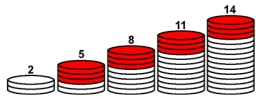Did you find an error or inaccuracy? Feel free to write us. Thank you!## Related math problems and questions:

• GP - three membersThe second and third of a geometric progression are 24 and 12(c+1), respectively, given that the sum of the first three terms of progression is 76. determine the value of c.
• Negative difference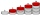Find four arithmetic progression members between 7 and -6.
• What areWhat are the four terms between 4 and 19?
• Sum of GP members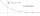Determine the sum of the GP 30, 6, 1.2, to 5 terms. What is the sum of all terms (to infinity)?
• Difference AP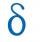Calculate the difference of arithmetic progression if the sum of its first 19 members Sn = 8075 and the first member is a1 = 20
• Geometric progressiobIf the sum of four consective terms of geometric progression is 80 and arithmetic mean of second and fourth term is 30 then find terms?
• InsertInsert five numbers between 8 and 27 such numbers that, with two given ones, they form the first seven members of the geometric sequence.
• Insert 3Insert five arithmetic progression members between -7 and 3/2.
• GP membersThe geometric sequence has 10 members. The last two members are 2 and -1. Which member is -1/16?
• Arithmetic progression 2The 3rd term of an Arithmetic progression is ten more than the first term, while the fifth term is 15 more than the second term. Find the sum of the 8th and 15th terms of the Arithmetic progression if the 7th term is seven times the first term.
• Common difference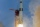The 4th term of an arithmetic progression is 6. If the sum of the 8th and 9th terms is -72, find the common difference.
• Find the 21Find the sum of the six terms of the finite geometric sequence 96, -48, 24, -12
• Fifth memberDetermine the fifth member of the arithmetic progression, if the sum of the second and fifth members equal to 73, and difference d = 7.
• Geometric progressionIn geometric progression, a1 = 7, q = 5. Find the condition for n to sum first n members is sn≤217.
• The sumThe sum of the first 10 members of the arithmetic sequence is 120. What will be the sum if the difference is reduced by 2?
• Finite arithmetic sequenceHow many numbers should be inserted between the numbers 1 and 25 so that all numbers create a finite arithmetic sequence and that the sum of all members of this group is 117?
• What are 2What are the two more terms of the GP a, ax, ax2, ax3, __, __?